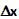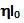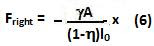## Sunday, July 4, 2010

### Irodov Problem 4.23The figure depicts the manner in which the mass m undergoes longitudinal oscillations in the spring. As the mass m is moves to the right, the right part of the spring is compressed while the left part of the spring expands. Thus, the left side of the spring pulls the mass to the left and the right part of the spring pushes the mass to the left.

The spring is an elastic material and follows the Young's law. In other words, if the Young's modulus of the springs material is, its area of cross section is A, then for any section of the spring we have,Let us first consider the entire spring. Lets its length be l0. Then, the restoring force resulting from a compression ofis given by,Hooke's law for springs says that,Comparing (2) and (3) we have,Now let us consider what happens when the mass m is pushed a distance x to the right.

The left part of the spring is expanded by an amount x. Since the length of the left part of the spring is, the restoring force for this part of the spring can be computed using (2) as,The right part of the spring is compressed by an amount x. Since the length of the right part of the spring is, the restoring force for this part of the spring can be computed using (2) as,Thus the net restoring force acting on the particle will be,From (7) it is clear that, the particle is undergoing simple harmonic motion with,